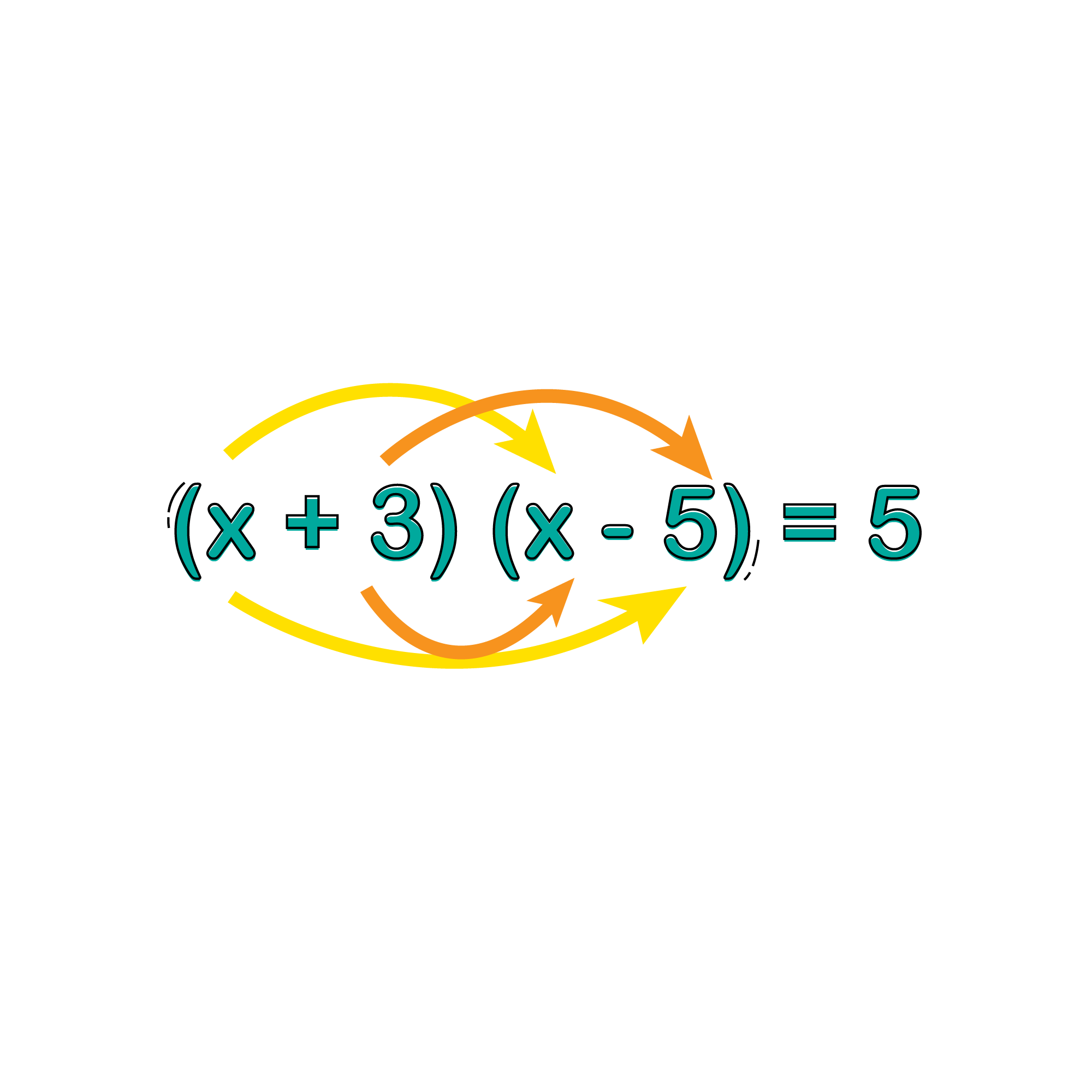SAT Math Algebra and Functions

The term quadratic comes from the Latin word quadratus meaning square because the variable gets squared (e.g., $$x^{2}$$ ). It is also called an equation of degree 2 because of the 2 superscripts on the x.

GENERAL OR STANDARD FORM: $$ax^{2}+bx+c=0$$

The following example illustrates the process for solving quadratic equations: Given that $$x^{2}+3x+8=12$$, what is x?

STEP 1: Move all the terms to the left side of the equation, combine them, and put them in the form $$ax^{2}+bx+c=0$$
Subtracting 12 from both sides of the equation, we get $$x^{2}+3x-4=0$$

STEP 2: In the above equation, you can see that b = 3 and c = -4. In order to factor this equation, you need to find two integers whose product is -4 and whose sum is 3. The only two integers that work are 4 and -1.

STEP 3: Rewrite the equation in the form (x + ?)(x + ?), where the question marks represent the two integers you solved for in the previous step.
$$x^{2}+3x-4=0$$
(x + 4)(x - 1) = 0

STEP 4: Since this product equals 0, one or both of the factors must be 0. In this problem, set each factor in parentheses independently to 0 and solve for x.
x + 4 = 0 OR x - 1 = 0
x = -4 OR x = 1

Thus, the two solutions or roots of the quadratic equation $$x^{2}+3x+8=12$$ are -4 and 1.

The SAT will often attempt to disguise quadratic equations by putting them in forms that do not quite look like the traditional form of $$ax^{2}+bx+c=0$$

Here is a very common “disguised” form for a quadratic: $$3w^{2}=6w$$

This is certainly a quadratic equation. However, it is very tempting to try to solve this equation without thinking of it as a quadratic. This classic mistake looks like this: $$3w^{2}=6w$$
3w = 6
w = 2

In solving this equation without factoring it like a quadratic, we have missed one of the solutions! Let us now solve it by factoring it as a quadratic equation: $$3w^{2}=6w$$
$$3w^{2}-6w=0$$
w(3w - 6) = 0
w = 0 or w = 2

In recognizing that $$3w^{2}=6w$$ is a disguised quadratic, we have found both solutions instead of accidentally missing one (in this case, the solution w = 0).

Here is another example of a disguised quadratic: Solve for b, given that $$\frac{36}{b}=b-5$$

Start by multiplying both sides of the equation by b. After you do this, you should recognize the components of a quadratic equation.
$$36=b^{2}-5b$$
$$b^{2}-5b-36=0$$
$$(b-9)(b+4)=0$$
Thus, b = 9 OR b = -4.

Some quadratics are hidden within more difficult equations, such as higher order equations (in which a variable is raised to the power of 3 or more). On the SAT, these equations can almost always be factored to find the hidden quadratic expression.

Example: Solve for x, given that $$x^{3}+2x^{2}-3x=0$$
$$x^{3}+2x^{2}-3x=0$$
$$x(x^{2}+2x-3)=0$$
x(x + 3)(x - 1) = 0

You have a product of three factors: x, (x + 3), and (x - 1). This product equals 0. Thus, one of the factors equals 0 That is, either: x = 0 OR x + 3 = 0 OR x - 1 = 0.

Be careful not just to divide both sides by x. You are only allowed to divide by a variable (or any expression) if you are absolutely sure that the variable or expression does not equal zero.

From this example, you can learn a general rule: If you have a quadratic expression equal to 0, and you can factor an x out of the expression, then x = 0 is a solution of the equation.

The vast majority of quadratic equations on the SAT can be solved by the factoring described earlier in this chapter. However, occasionally you might need to use the quadratic formula, which can solve any quadratic equation but is cumbersome to use. At AP Guru, we only recommend that you use the formula if the factorization method does not work.

QUADRATIC FORMULA: For any quadratic equation $$ax^{2}+bx+c=0$$ the solutions for x are $$x=\frac{-b\pm \sqrt{b^{2}-4ac}}{2a}$$

Example: If $$x^{2}+8x+13=0$$, what is x?

You can find the solutions simply by plugging the coefficients from the equation into the quadratic formula: $$x=\frac{-8\pm \sqrt{8^{2}-4(1)(13)}}{2(1)}$$
$$x=\frac{-8\pm \sqrt{64-52}}{2}$$
$$x=-4+\sqrt{2}\; or\; -4-\sqrt{2}$$

The expression underneath the radical in the formula $$(\sqrt{b^{2}-4ac})$$ is called the DISCRIMINANT and determines the nature of the ROOTS (the solutions of the quadratic equation)

#### Nature of roots:

• $$b^{2}-4ac=0$$ Real and equal. 2 Solutions
• $$b^{2}-4ac>0$$ Real but unequal. 1 Solution
• $$b^{2}-4ac$$ No Solution